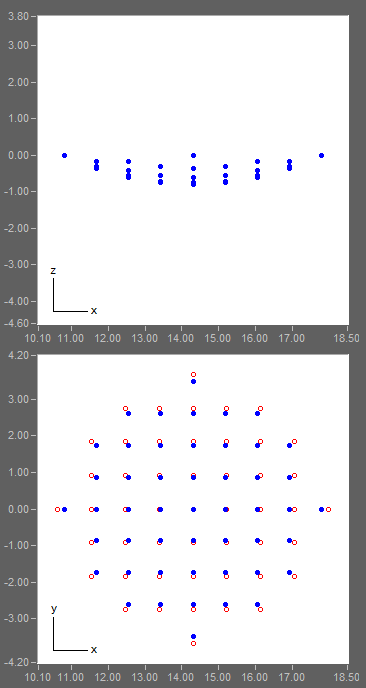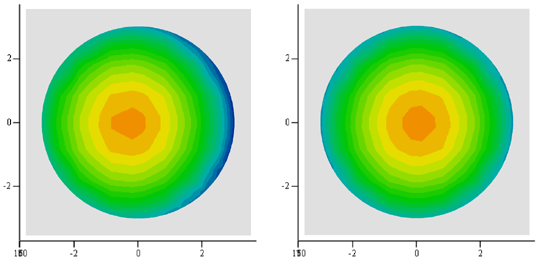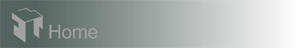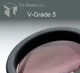Case 5: Spherical Surface Mapping - Planetary

To obtain a thickness distribution on a 3-dimensional surface, points on an X-Y grid (or any other arrangement) can be entered in the "Surface Coated" panel.

In this example, we examine the thickness distribution on a spherical and convex lens surface from the same model planetary rotation coater. A total of 49 surface points, shown below in X-Z and X-Y views, are computed for a thickness contour plot. (The XYZ coordinate system is fixed on the rotating fixture; the red circles indicate the orientations of the surface normal vectors.)

First, we leveled the lens in the plane of the fixture, the center of the lens being 14.3 cm from the center of the fixture. The resultant thicknesses for the 49 surface points are utilized to create the contour plot shown in the left column below. The thickness distribution exhibits a significant asymmetry to the center of the lens.

To correct the asymmetry, we can pivot the lens slightly. It is found that a pivot angle of -2.6 degrees yields the most symmetric distribution, shown in the right column. The overall peak-to-valley nonuniformity for the leveled lens and the pivoted lens is 7.6% and 6.5%, respectively.Leveled lens
Pivoted lensmaximum=171.15; minimum=150.51
maximum=170.96; minimum=146.59Hardware requirements
Request a Free Trial